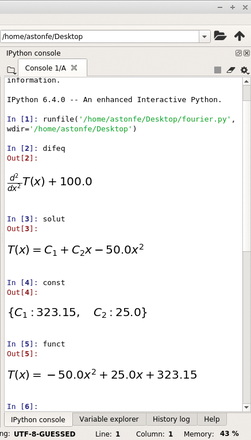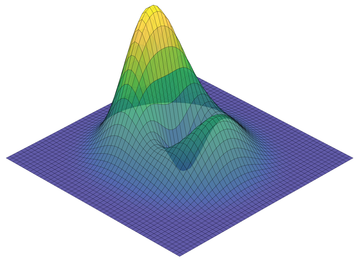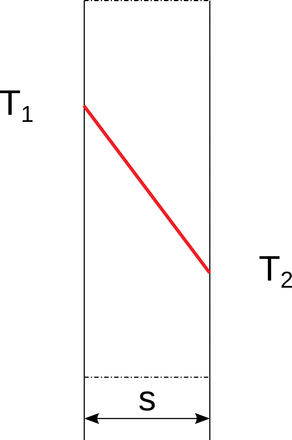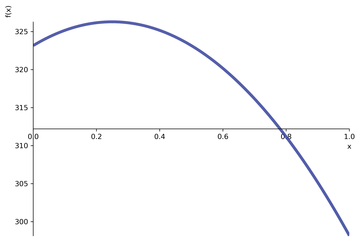# Complex Math

The Python SymPy library for symbolic mathematics allows you to create complex mathematical expressions.

SymPy  is a computer algebra system written in the Python programming language. Among its many features are algorithms for computing derivatives, integrals, and limits; functions for manipulating and simplifying expressions; functions for symbolically solving equations and ordinary and partial differential equations; two- and three-dimensional (2D and 3D) plotting; and much more (e.g., see the SymPy features list  and Table 1 in a PeerJ Computer Science paper ).

Table 1

symbols Assignment

 Expression Description Comma-delimited arguments Space-delimited arguments Colon-delimited range Range assignment Assigned with a different name Greek letters Long names

In this article, I use SymPy first for an algebraic function and then for the Fourier equation to explore heat conduction calculations. All the examples presented here use the Spyder 3.2.8 Python development environment (Anaconda 5.2.0) on Mint 19.0 Xfce. Because the full code is too long for a printed article, it's freely available on GitHub . Python's reserved words are not classified (e.g., as in Scilab), so I use "command" as a synonym of "reserved word."

#### Algebraic Function

To carry out an algebraic calculation, you must define symbolic variables with the `symbols` command, which returns a sequence of symbols with names taken from the `names` argument (`def symbols(names, args):`), which can be a comma- or whitespace-delimited string or a string delimited in other ways (Table 1) . Symbols also can define a parametric curve – for example, u and v are the symbols and x , y , z are the coordinates, as f (u ,v ).

According to the SymPy documentation, to view well-rendered equations, use the `init_printing()` command in your script, which will automatically enable the best printer available in your environment (i.e., LaTeX, if previously installed). The rendering is very good even if LaTeX is not installed (Figure 1).Figure 1: This Spyder screenshot shows how well equations are rendered, even when LaTeX is not installed.

Consider the algebraic function:

`def f(x): return x/(x**2+1)`

According to Table 2, the `roots` are the x -coordinates of the minimum and maximum points, respectively. The two corresponding y -coordinates are then calculated by:

```ymin=f(x).subs(x,const)
ymax=f(x).subs(x,const)```

Table 2

SymPy Commands

 Operation Calculation View Integral `integ=integrate(f(x),x)` `integ` + Enter First derivative `deriv=diff(f(x),x)` `deriv` + Enter Roots `roots=solve(deriv)` `roots` + Enter 2D plot – `plot(f(x),(x,xmin,xmax))` 3D plot – `plot3d(f,(x,xmin,xmax),(y,ymin,ymax))`

Use `ymin,ymax`+Enter to view the result. Another way to calculate the two x -coordinates is with the use of `scipy.optimize.fmin`:

```from scipy.optimize import fmin
xmin=fmin(f,0)
xmax=fmin(lambda x: -f(x),0)```

The indefinite integral is simply calculated as shown in Table 2. For the definite integral, you must specify the limits, for example,

`integ=integrate(f(x),(x,xmin,xmax))`

in which `xmin` and `xmax` are the interval limits. The definite integrals also can be calculated with `scipy.integrate.quad`. The `plot` command has the `adaptive` option set to `True` by default, so the resulting plot is segmented. If `adaptive` is set to `False`, it's possible to specify the number of points (`nb_of_points`) (Figures 2 and 3). Figure 4 shows the plot of a 3D function.Figure 4: A 3D plot of f=(x**2+2.5*y**2-y)*exp(1-(x**2+y**2)).

The most important thing to remember about `plot3d` is that `_backend.ax.collections` must be called first to set line color, color map, and alpha values. I have used the `parula` color map built from the `parula.csv` data . The complete scripts for these first two examples are `function2d.py` and `function3d.py`, respectively .

The `mpmath.splot` and `plot3d_parametric_surface` functions plot parametric surfaces. For example, a corkscrew surface (Figure 5) is defined and plotted by the code:

```u,v=symbols("u,v")
x=cos(u)*(cos(v)+3)
y=sin(u)*(cos(v)+3)
z=sin(v)+u
plot3d_parametric_surface(x,y,z,(u,umin,umax),(v,vmin,vmax),...)```

The complete script for this example is `corkscrew.py` .

#### Flat Wall in Steady State

The Fourier equation is an example of a second-order (parabolic) partial differential equation (PDE). This equation is the fundamental tool for the study of thermal fields inside bodies. In practice, the Fourier equation integration is possible only in few cases, with a drastic simplification of the hypotheses. Two of these cases, important for technical applications, are examined in this section.

Consider a flat, homogeneous, infinite wall of constant thickness s (Figure 6) with faces of uniform temperatures in steady state (i.e., time independent). In this first case, the Fourier equation is:Figure 6: A flat wall section of thickness s with faces of uniform temperatures.

where `x`=0 is the left side and `x`=s is the right side of the section. The `plot` command shows the linear relationship between T and x within the wall section (Figure 6, red line).

With internal heat generation, the Fourier equation becomes

in which `H` is the volumetric heat generation and lambda is the thermal conductivity of the wall. In this second case, the `plot` command shows the temperature in the wall as a parabolic trend (Figure 7). In both cases, the temperature trend is calculated by:

```solut=dsolve(difeq,T(x))
const=solve([solut.rhs.subs(x,0)-T1,solut.rhs.subs(x,s)-T2])
funct=solut.subs(const)```Figure 7: Temperature trend within the wall section with internal heat generation.

The differential equation is solved by `dsolve` in the first line, the constants are calculated using its right-hand side (`rhs`) in the second line, and the final function is calculated in the third line. The `funct` function is plotted in the range from `x`=0 to `x`=s through the wall section. The complete script is `fourier.py` . To specify the boundary conditions, it's also possible to use `dsolve` with the `ics={...}` option, in which `ics` means initial conditions. At the present time, this option works only on particular cases, so it's not for a general use.

#### Conclusions

The SymPy library has very interesting symbolic manipulation capabilities, only a few of which I demonstrated here. With SymPy you can create many other, much more complex, expressions. In addition to the references already mentioned, you will find an interesting introduction to the Python language for science and engineering in Chapter 12 (dedicated to SymPy) of an online book . Additionally, you can purchase a nice book on differential equations  at your favorite bookstore. Although that author used another well-known free and open source computer algebra system called Maxima , as well as various other commercial software, for the examples in his book, you can check out his comprehensive online SymPy tutorials  . I hope this article will stimulate your curiosity in this computer algebra system for complex mathematics.

Infos

1. SymPy: https://www.sympy.org/en/index.html
2. SymPy features: https://www.sympy.org/en/features.html
3. Meurer, A., C. P. Smith, M. Paprocki, et al. 2017. SymPy: Symbolic computing in Python. PeerJ Computer Science 3:e103. doi:10.7717/peerj- cs.103. https://peerj.com/articles/cs-103.pdf. Last accessed 17 October 2018
4. Code on GitHub: https://github.com/astonfe/sympy
5. Humpherys, J., and T. J. Jarvis (eds.). 2018. "Introduction to SymPy" In: Labs for Foundations of Applied Mathematics: Python Essentials , Chapter 9, http://www.acme.byu.edu/2018-2019-materials (click on PythonEssentials.pdf ). Last accessed 14 October 2018
6. Fangohr, H. 2016. Python for Computational Science and Engineering . http://www.southampton.ac.uk/~fangohr/teaching/python/book.html. Last accessed 14 October 2018
7. Dobrushkin, V. A. 2014. Applied Differential Equations . Boca Raton, Florida: CRC Press, 731 pp.
8. Maxima: http://maxima.sourceforge.net
9. "SymPy Tutorial 1" by Vladimir Dobrushkin, http://www.cfm.brown.edu/people/dobrush/am33/SymPy/index.html
10. "SymPy Tutorial 2" by Vladimir Dobrushkin, http://www.cfm.brown.edu/people/dobrush/am34/SymPy/index.html @IE

The Author

Stefano Rizzi works in the areas of analytical chemistry and textile chemistry. He has been a Linux user since 1998.

Express-Checkout as PDF
Price \$2.95
(incl. VAT)